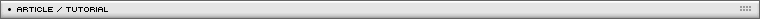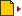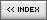The art of scriptable skew
[ July 13, 2004 ] by Eric Lin
Basic 3D in Flash: 1/5
In this first article, the author explains how to skew movieclips, the basis to create 3D games.

Flash MX add a function to distort "shapes"; it is only a "shape" that we can distort. We still can not distort "movieClip" or "bitmap". So, when we need to skew a movieClip, we can only skew it into a regular diamond (quandrangle);  no perspective skew can be applied to movieClip either manually or by script, unless the target is a "shape" or something you break-apart into shape.

To distort a movieClip, we need a trick to make the picture by multiple stripes and control them respectively. However, it is not practical for large movieClip. If a movieClip has 300 width, and we make a stripe 3 pixels wide, we get 100 movieClips to construct the original movieClip. The CPU will go mad. Below is a movie demonstrate the principles.

Isn't there any way? Could we break them into two triangle and distort and skew each triangle and combine them together? I tried this by many ways. After I think deep about the math, I get a conclusion: it is not possible.
Below is a movie I did. I made two triangle to stitch them together. At first glance, the distortion skew seems not too bad. But if we click the button, we will realize that, it is not an even distortion. The stitch line will be obvious sometimes.

HOW TO DINAMICALLY SKEW A MOVIECLIP?

The common way is make a 180 or 360 frame tween to render the skew angle. The pitfall is that it is not accurate enough. If we want to construct 3D object by multiple skewing face, we can not get a seamless model without crack. So, could we use math to skew a movieClip? Here is the secret.

Skewing can be obtained easily by puting movieclip in a stretched movieClip. MovieClip itself does rotation and _parent does stretching. Below is the swf showing a skew of 100x100 square.

The _parent can also do rotation and the movieClip can also do stretch. With various combination of stretch and rotation we can make the square movieClip skew to a shape that we want. But, how do we know how much _rotation and _xscale and _yscale we should apply to _parent and movieClip itself ? I will explain to you.

Ok, we can first stretch our movieClip into 100x100 size and then rotate -45 degrees. That will be what we see below. Now try to control 3 parameters: the _parent._yscale, and the _xscale and _yscale of movieClip itself. You can click the buttons to increase and decrease the value. I suggest you to observe the change of _parent._yscale first. That is the core of the skew math. You will realize that, you can easily make a skew with the angle you want and the shape you want. After you get it, we will discuss the math.

Here is the diagram about the math to calculate the relation ship of skew angle and _parent._yscale; I don't want to explain them in details. You should try to develop the math equation yourself. Anyway, stretchig of _parent._yscale will increase the skew angle and shrink the _parent.yscale will shapen the skew angle. If you figure out this, you will know it is very simple math.

Ok, we go step by step to make a a movieClip of 200*100 to conform to the 3 draggable corners (you can not change the 4th corner, because Flash allows only a diamond skew. As said before, we can't do distortional skew such as perspective skew).

These are the steps. First, we calculate the skew angle: Angle(P2-P0-P1); we make a quadrangle with that angle; then we stretch the arm of "width" and then stretch the arm of "height". Then we put the prepared quadrangle to the place we want it to be, setting _x, _y and _rotation.

Before the function can be applied, some preparation should be done. First, the movieClip that is going to do skew must have its registration point at left-top corner, not at the center of movieClip. Create an empty holder movieClip, and put this movieClip in it. The registration point should be the (0,0) point. Now give your movieClip an instance name of "mc". If you prefer to give a name you like, for example "mySkewableMC", then in the first frame of this holder movieClip, write this.mc=mySkewableMC.
The above setting is just for my skew function. You can develop your own skew function equation and modify it.

The function is:

```function skewObj (obj, mcW, mcH, pt0, ptH, ptW)
{
function distance (pt1, pt2)
{
var dy = pt2.y-pt1.y;
var dx = pt2.x-pt1.x;
var side = Math.sqrt(dy*dy+dx*dx);
return side;
}
obj._x = pt0.x;
obj._y = pt0.y;
obj._yscale = 100;
var angleP2 = Math.atan2(ptW.y-pt0.y, ptW.x-pt0.x);
var angleP1 = Math.atan2(ptH.y-pt0.y, ptH.x-pt0.x);
var dAngle = (angleP1-angleP2)/2;
var arm = Math.sqrt(2)/2/Math.cos(dAngle);

// original a 100x100 model, now use 1x1 model
obj._rotation = (180/Math.PI)*(angleP1-dAngle);
obj.mc._rotation = -45;
obj._yscale = 100*Math.tan(dAngle);
obj.mc._xscale = distance(ptW, pt0)*100/arm/mcW;
obj.mc._yscale = distance(ptH, pt0)*100/arm/mcH;```

Here, obj is the instance name of your holder movieClip. mcW and mcH are the original width and height of the  movieClip inside the holder. pt0, ptH and ptW are point object with the format of {x:??,y:??}; they are in the same coordinate system of the holder movieClip.

Ok, below is an example to make a movieClip stretched by 3 draggable corners movieClip p0, pH, pW.

```H = obj.mc._height;
W = obj.mc._width;
{
pt0 = {x:p0._x, y:p0._y};
ptH = {x:pH._x, y:pH._y};
ptW = {x:pW._x, y:pW._y};
skewObj(obj, W, H, pt0, ptH, ptW);
}